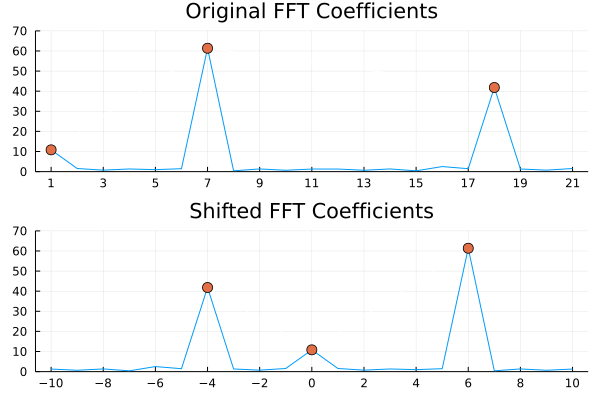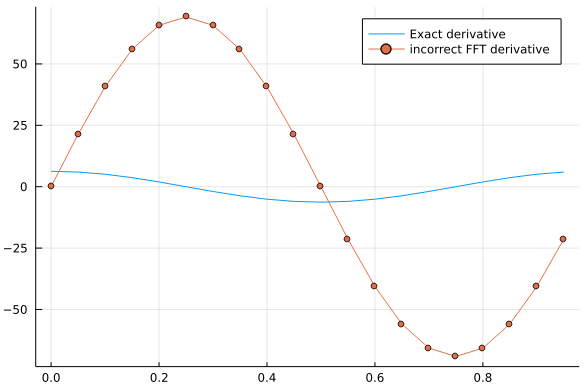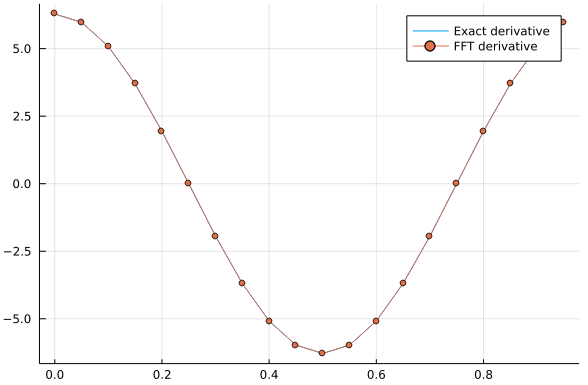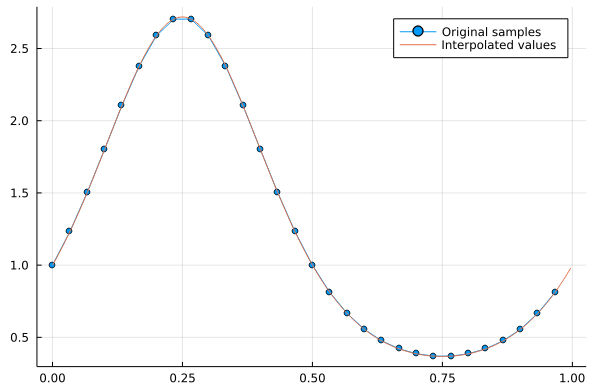# Using the Fast Fourier Transform

In this post, we will cover the basics of getting FFTs up and running, plus one basic performance tip. Additionally, we will go trough the usual nitty-gritty of FFTs: coefficient orders, normalization constants, and aliasing. We will illustrate this with some code examples about FFT-differentiation and FFT-interpolation.

## Abstract FFTs and FFTW

Julia implements FFTs according to a general Abstract FFTs framework. That framework then relies on a library that serves as a backend. In case we want to use the popular FFTW backend, we need to add the FFTW.jl package.

using FFTW


Intel’s MKL provides a notable implementation, which is available in Julia via the same FFTW.jl package. To enable it, simply open the Julia REPL and type:

FFTW.set_provider!("mkl")


After restarting Julia and running ‘using FFTW’ again, the required MKL components will be automatically downloaded and installed.

## Coefficient Order and Normalization

The Abstract FFTs defines both the DFT and inverse DFT using two formulas which I took me a while to restate in more familiar terms:

$$\mathrm{DFT}(A)[k] = \sum_{n=1}^{\mathrm{length(A)}} \mathrm{exp}\left( -i \frac{2\pi(n-1)(k-1)}{\mathrm{length(A)}}\right) A[n]$$ $$\mathrm{IDFT}(A)[k] = \frac{1}{\mathrm{length(A)}} \sum_{n=1}^{\mathrm{length(A)}} \mathrm{exp}\left( +i \frac{2\pi(n-1)(k-1)}{\mathrm{length(A)}}\right) A[n]$$

To interpret these formulas, I think it’s useful to define an N-point grid in $$[0,2\pi)$$, that is, starting from zero and omitting the end-point.

$$\lbrace x_j \rbrace_{0 \le j \le N-1} = \left\lbrace \frac{2\pi j}{N} : \quad 0 \le j \le N-1 \right\rbrace$$

Given a vector $$v = \lbrace v_j \rbrace_{0 \le j \le N-1}$$, we see that the DFT defined above will compute:

$$\mathrm{DFT}(v)[k] = \sum_{j=0}^{N-1} e^{-ik x_j}v_j \qquad 0 \le k \le N-1$$

and the corresponding IFFT of a vector v yields

$$\mathrm{IDFT}(v)[j] = \frac{1}{N} \sum_{k=0}^{N-1} e^{ik x_j}v_{k} \qquad 0 \le j \le N-1$$

## Aliasing and Negative Frequencies

The original FFT frequencies appear to be

$$k = 0, \dots, N-1$$

But there is a tricky thing going on behind the scenes, called ‘aliasing’, which is a consequence of the simple fact that, for an N-point grid such as ours, we have the following identity

$$e^{i N m x_j} = 1 \qquad m \in \mathbf{Z}$$

This implies, in particular, that Fourier modes with frequencies above $$N/2$$ (also refered to as the ‘Nyquist frequency’) are equivalent, in our finite grid, to negative-frequency modes with $$k > -N/2$$.

In many cases, it becomes necessary to remove frequencies above Nyquist, and restate the IDFT in the more convenient form

$$\frac{1}{N} \sum_{k=-N/2+1}^{N/2} e^{ik x_j}w_k \quad j = 1,…,N.$$

However, to do this, the original FFT coefficients $$v_k$$ must be reordered into the $$w_k$$ coefficients illustrated in the above formula. This reordering is handled with the fftshift function.

## fftshift and fftfreq

The fftshift function reorders a vector in a way that can be illustrated by the following two examples, for N even and odd.

fftshift(1:10)'

 6  7  8  9  10  1  2  3  4  5

fftshift(1:11)'

 7  8  9  10  11  1  2  3  4  5  6


Another useful function, fftfreq gives the correct frequencies (that is, below Nyquist) that the FFT algorithm is producing, divided by N.

N=10
fftfreq(N)'*N

 0.0  1.0  2.0  3.0  4.0  -5.0  -4.0  -3.0  -2.0  -1.0

N=11
fftfreq(N)'*N

 0.0  1.0  2.0  3.0  4.0  5.0  -5.0  -4.0  -3.0  -2.0  -1.0


### Original and Shifted FFTs

We can use the fftshift and fftfreq functions to create the shifted FFT and be able to tell which frequency corresponds to each coefficient.

To do this, we apply the function fftshift to both the fft coefficients, and to the original (below Nyquist) frequencies returned by fftfreq.

using FFTW
using plots

N = 21
xj = (0:N-1)*2*pi/N
f = 2*exp.(17*im*xj) + 3*exp.(6*im*xj) + rand(N)

original_k = 1:N
shifted_k = fftshift(fftfreq(N)*N)

original_fft = fft(f)
shifted_fft = fftshift(fft(f))

p1 = plot(original_k,abs.(original_fft),title="Original FFT", xticks=original_k[1:2:end], legend=false, ylims=(0,70));
p1 = plot!([1,7,18],abs.(original_fft[[1,7,18]]),markershape=:circle,markersize=6,linecolor="white");
p2 = plot(shifted_k,abs.(shifted_fft),title="Shifted FFT",xticks=shifted_k[1:2:end], legend=false, ylims=(0,70));
p2 = plot!([-4,0,6],abs.(shifted_fft[[7,11,17]]),markershape=:circle,markersize=6,linecolor="white");
plot(p1,p2,layout=(2,1))## FFT derivative

In many applications, we want to take advantage that the derivative operator is transformed into a multiplication operator in Fourier space. By applying a subsequent inverse transform, we can expect to obtain the derivative of a function using FFTs, using the well-known relation:

$$\mathcal{F}[g’](\xi) = \frac{2\pi}{L} \xi \mathcal{F}[g](\xi)$$

However, this is not so simple to translate into the discrete setting.

For example, let’s compute the FFT-derivative of a periodic function defined in $$(0,L)$$. The following code yields an incorrect result:

using FFTW
using plots

N = 20;
L = 1;
xj = (0:N-1)*L/N;
f = sin.(2*pi*xj)
df = 2*pi*cos.(2*pi*xj)

k = 1:N
df_fft = ifft( 2*pi/L * k.* fft(f) )

plot(xj,real(df),label="Exact derivative")
plot!(xj,real(df_fft),label="incorrect FFT derivative",markershape=:circle)The reason this doesn’t work is related to the previous section about aliasing. Even though, in our grid, we have

$$\sin(5 x_j) = \frac{e^{-5 i x_j} + e^{5 i x_j}}{2i} + \frac{e^{15 i x_j} + e^{5 i x_j}}{2i}$$

Clearly, the frequency corresponding to k=15 should not be employed when computing the derivative, and that is actually what the above code is doing.

The correct derivative algorithm uses the negative coefficients mentioned in the previous section.

In order to obtain the correct result, we to correct these two lines:

k = fftfreq(N)*N;
df_fft = ifft( 2*pi*im/L * k.* fft(f) );## FFT interpolation

FFT interpolation is based on adding zeros at the end of the Fourier coefficient vector. In such a way, the inverse FFT will produce more output, using the same (non-zero) Fourier coefficients. When implementing this procedure, it’s important to pay attention to the interplay of the normalization constants, the number of points, and the interpolation grid that is being employed.

Let’s consider a function in $$[0,1]$$ consisting of $$N$$ random values, which we want to interpolate into $$Ni$$ points.

The following simple test illustrates that.

using Plots
using FFTW

N = 30;
x = (0:N-1)/N;

Ni = 300;
xi = (0:Ni-1)/Ni;

f = rand(N);
f_interp = real(ifft( [fft(f); zeros(Ni-N)] )) *Ni/N ;

plot(x,f, label="Original samples",markershape=:circle)
plot!(xi,f_interp,label="Interpolated values")## Precomputing FFT Plans

In case we are going to perform several FFTs of the same size, it is convenient to pre-compute the so-called ‘fft plans’.

The most general (complex-valued) of these functions are: plan_fft, and plan_ifft. An example on how to use plan_fft is:

x=rand(ComplexF64,1000);
p = plan_fft(x; flags=FFTW.ESTIMATE, timelimit=Inf)


As for timings, we can check out that preparaing the plan in this case has produce a 3X speed-up over the basic command.

@time fft_x = p*x;

  0.000029 seconds (2 allocations: 31.500 KiB)

@time fft_x = fft(x);

  0.000093 seconds (34 allocations: 33.922 KiB)


In-place FFTs (that is, an FFT that modifies the input vector rather than allocating memory and returning a new variable) can be optimized similarly, as well, by doing

p = plan_fft!(x)